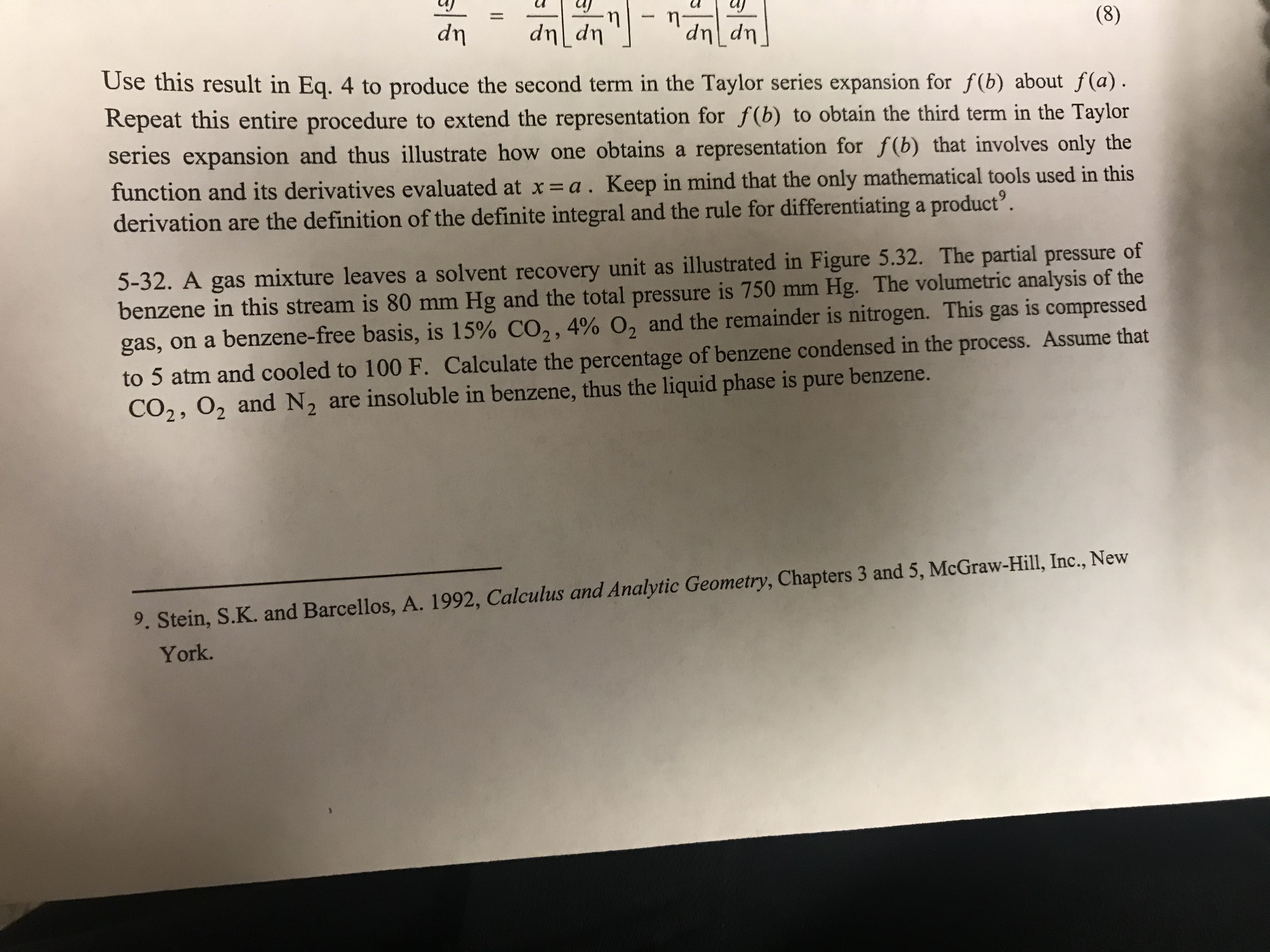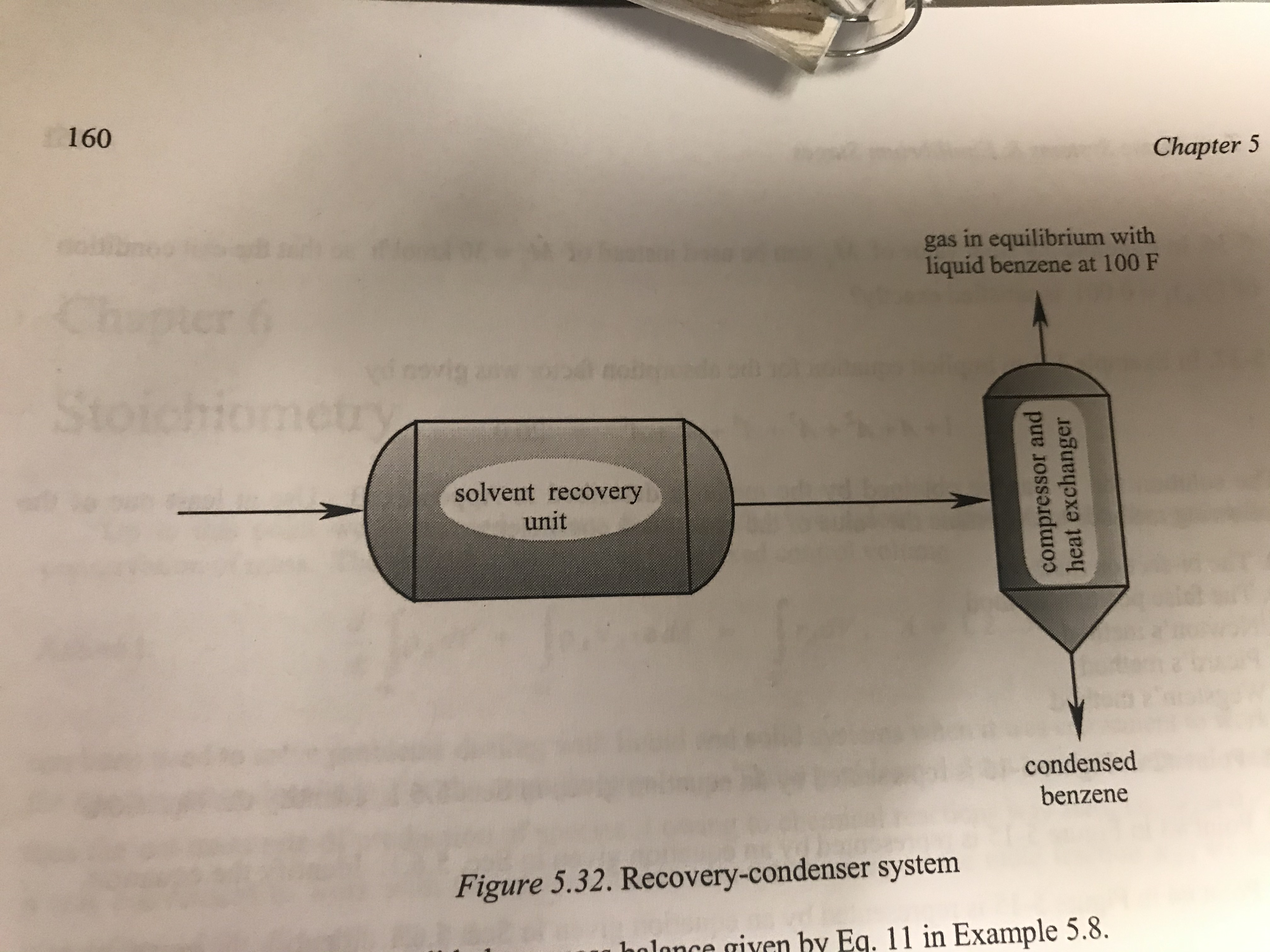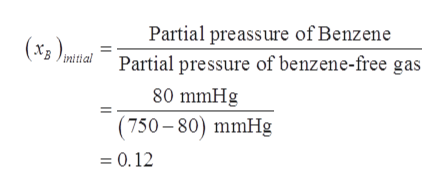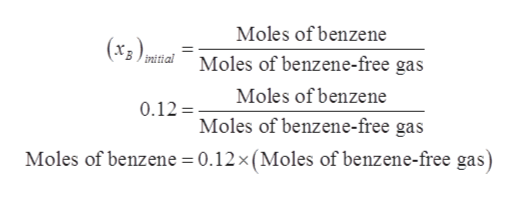# 17(8)up TupUse this result in Eq. 4 to produce the second term in the Taylor series expansion for f(b) about f(a)dnup TupRepeat this entire procedure to extend the representation for f(b) to obtain the third term in the Taylorseries expansion and thus illustrate how one obtains a representation for f(b) that involves only thefunction and its derivatives evaluated at x=a . Keep in mind that the only mathematical tools used in thisderivation are the definition of the definite integral and the rule for differentiating a product'.5-32. A gas mixture leaves a solvent recovery unit as illustrated in Figure 5.32. The partial pressure ofbenzene in this stream is 80 mm Hg and the total pressure is 750 mm Hg. The volumetric analysis of thegas, on a benzene-free basis, is 15% CO,, 4% O, and the remainder is nitrogen. This gas is compressed2to 5 atm and cooled to 100 F. Calculate the percentage of benzene condensed in the process. Assume thatCO2, 02 and N2 are insolub le in benzene, thus the liquid phase is pure benzene.9. Stein, S.K. and Barcellos, A. 1992, Calculus and Analytic Geometry, Chapters 3 and 5, McGraw-Hill, Inc., NewYork. compressor andheat exchanger160Chapter 5gas in equilibrium withliquid benzene at 100 Fcrwovig>4solvent recoveryunitcondensedbenzeneFigure 5.32. Recovery-condenser systemby Eq. 11 in Example 5.8.

Question
14 viewshelp_outlineImage Transcriptionclose17 (8) up Tup Use this result in Eq. 4 to produce the second term in the Taylor series expansion for f(b) about f(a) dn up Tup Repeat this entire procedure to extend the representation for f(b) to obtain the third term in the Taylor series expansion and thus illustrate how one obtains a representation for f(b) that involves only the function and its derivatives evaluated at x=a . Keep in mind that the only mathematical tools used in this derivation are the definition of the definite integral and the rule for differentiating a product'. 5-32. A gas mixture leaves a solvent recovery unit as illustrated in Figure 5.32. The partial pressure of benzene in this stream is 80 mm Hg and the total pressure is 750 mm Hg. The volumetric analysis of the gas, on a benzene-free basis, is 15% CO,, 4% O, and the remainder is nitrogen. This gas is compressed 2 to 5 atm and cooled to 100 F. Calculate the percentage of benzene condensed in the process. Assume that CO2, 02 and N2 are insolub le in benzene, thus the liquid phase is pure benzene. 9. Stein, S.K. and Barcellos, A. 1992, Calculus and Analytic Geometry, Chapters 3 and 5, McGraw-Hill, Inc., New York. fullscreenhelp_outlineImage Transcriptionclosecompressor and heat exchanger 160 Chapter 5 gas in equilibrium with liquid benzene at 100 F cr wovig >4 solvent recovery unit condensed benzene Figure 5.32. Recovery-condenser system by Eq. 11 in Example 5.8. fullscreen
check_circle

Step 1

Vapor pressure of benzene in gas mixture before compression is given as 80 mmHg.

Total pressure of the gas mixture before compression is given as 750 mmHg.

Mole fraction of benzene in the gas mixture before compression on benzene-free basis will be:help_outlineImage TranscriptionclosePartial preassure of Benzene Partial pressure of benzene-free gas (x3 ) initial 80 mmHg (750-80) mmHg =0.12 fullscreen
Step 2

This can also be written as:help_outlineImage TranscriptioncloseMoles of benzene (x3)mitaMoles of benzene-free gas Moles of benzene 0.12= Moles of benzene-free gas Moles of benzene 0.12 x (Moles of benzene-free gas) fullscreen
Step 3

When this gas is cooled and compressed  to 100  ̊F and 5 atm (3800 mmHg) , liquid...

### Want to see the full answer?

See Solution

#### Want to see this answer and more?

Solutions are written by subject experts who are available 24/7. Questions are typically answered within 1 hour.*

See Solution
*Response times may vary by subject and question.
Tagged in

### Process Calculations